# Logistic Regression¶

## Agenda¶

1. Refresh your memory on how to do linear regression in scikit-learn
2. Attempt to use linear regression for classification
3. Show you why logistic regression is a better alternative for classification
4. Brief overview of probability, odds, e, log, and log-odds
5. Explain the form of logistic regression
6. Explain how to interpret logistic regression coefficients
7. Demonstrate how logistic regression works with categorical features
8. Compare logistic regression with other models

## Part 1: Predicting a Continuous Response¶

In :
# glass identification dataset
import pandas as pd
url = 'http://archive.ics.uci.edu/ml/machine-learning-databases/glass/glass.data'
col_names = ['id','ri','na','mg','al','si','k','ca','ba','fe','glass_type']
glass = pd.read_csv(url, names=col_names, index_col='id')
glass.sort('al', inplace=True)
glass.head()

Out:
ri na mg al si k ca ba fe glass_type
id
22 1.51966 14.77 3.75 0.29 72.02 0.03 9.00 0 0.00 1
185 1.51115 17.38 0.00 0.34 75.41 0.00 6.65 0 0.00 6
40 1.52213 14.21 3.82 0.47 71.77 0.11 9.57 0 0.00 1
39 1.52213 14.21 3.82 0.47 71.77 0.11 9.57 0 0.00 1
51 1.52320 13.72 3.72 0.51 71.75 0.09 10.06 0 0.16 1

Question: Pretend that we want to predict ri, and our only feature is al. How could we do it using machine learning?

Answer: We could frame it as a regression problem, and use a linear regression model with al as the only feature and ri as the response.

Question: How would we visualize this model?

Answer: Create a scatter plot with al on the x-axis and ri on the y-axis, and draw the line of best fit.

In :
import seaborn as sns
import matplotlib.pyplot as plt
%matplotlib inline
sns.set(font_scale=1.5)

In :
sns.lmplot(x='al', y='ri', data=glass, ci=None)

Out:
<seaborn.axisgrid.FacetGrid at 0x4136358>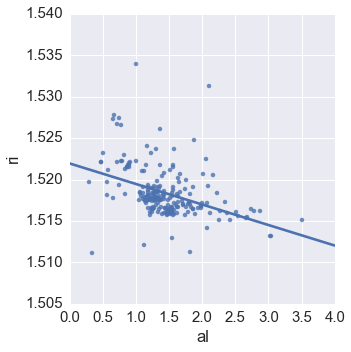Question: How would we draw this plot without using Seaborn?

In :
# scatter plot using Pandas
glass.plot(kind='scatter', x='al', y='ri')

Out:
<matplotlib.axes._subplots.AxesSubplot at 0x18395d30>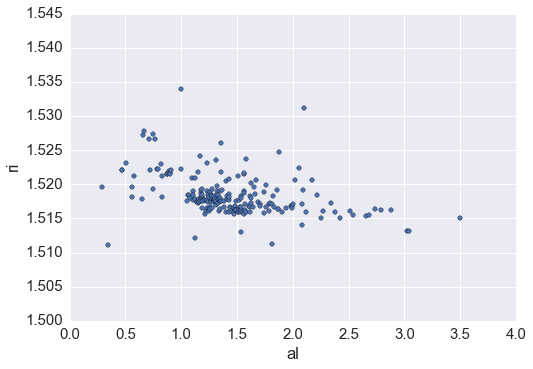In :
# equivalent scatter plot using Matplotlib
plt.scatter(glass.al, glass.ri)
plt.xlabel('al')
plt.ylabel('ri')

Out:
<matplotlib.text.Text at 0x187b42b0>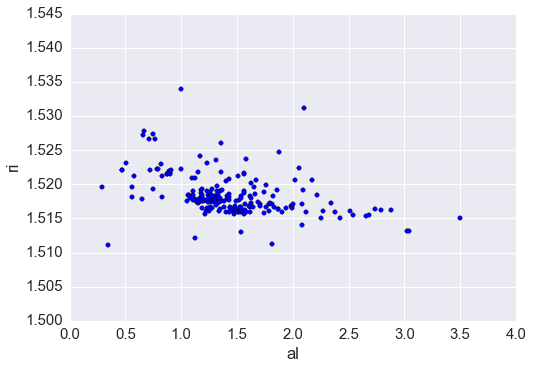In :
# fit a linear regression model
from sklearn.linear_model import LinearRegression
linreg = LinearRegression()
feature_cols = ['al']
X = glass[feature_cols]
y = glass.ri
linreg.fit(X, y)

Out:
LinearRegression(copy_X=True, fit_intercept=True, n_jobs=1, normalize=False)
In :
# make predictions for all values of X
glass['ri_pred'] = linreg.predict(X)
glass.head()

Out:
ri na mg al si k ca ba fe glass_type ri_pred
id
22 1.51966 14.77 3.75 0.29 72.02 0.03 9.00 0 0.00 1 1.521227
185 1.51115 17.38 0.00 0.34 75.41 0.00 6.65 0 0.00 6 1.521103
40 1.52213 14.21 3.82 0.47 71.77 0.11 9.57 0 0.00 1 1.520781
39 1.52213 14.21 3.82 0.47 71.77 0.11 9.57 0 0.00 1 1.520781
51 1.52320 13.72 3.72 0.51 71.75 0.09 10.06 0 0.16 1 1.520682
In :
# plot those predictions connected by a line
plt.plot(glass.al, glass.ri_pred, color='red')
plt.xlabel('al')
plt.ylabel('Predicted ri')

Out:
<matplotlib.text.Text at 0x1a1fbda0>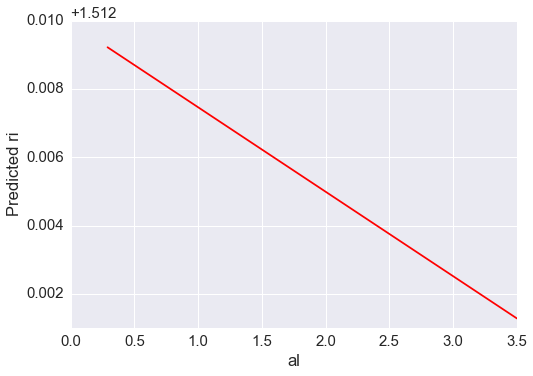In :
# put the plots together
plt.scatter(glass.al, glass.ri)
plt.plot(glass.al, glass.ri_pred, color='red')
plt.xlabel('al')
plt.ylabel('ri')

Out:
<matplotlib.text.Text at 0x1a21d7b8>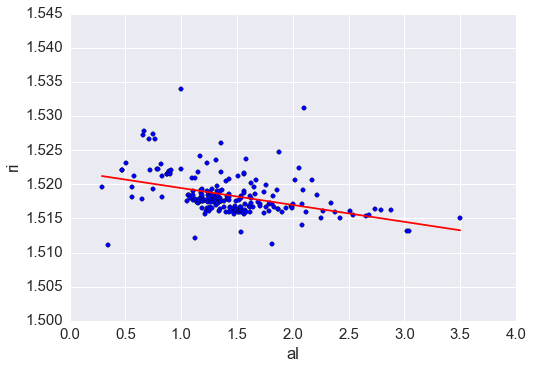### Refresher: interpreting linear regression coefficients¶

Linear regression equation: $y = \beta_0 + \beta_1x$

In :
# compute prediction for al=2 using the equation
linreg.intercept_ + linreg.coef_ * 2

Out:
array([ 1.51699012])
In :
# compute prediction for al=2 using the predict method
linreg.predict(2)

Out:
array([ 1.51699012])
In :
# examine coefficient for al
zip(feature_cols, linreg.coef_)

Out:
[('al', -0.002477606387469623)]

Interpretation: A 1 unit increase in 'al' is associated with a 0.0025 unit decrease in 'ri'.

In :
# increasing al by 1 (so that al=3) decreases ri by 0.0025
1.51699012 - 0.0024776063874696243

Out:
1.5145125136125304
In :
# compute prediction for al=3 using the predict method
linreg.predict(3)

Out:
array([ 1.51451251])

## Part 2: Predicting a Categorical Response¶

In :
# examine glass_type
glass.glass_type.value_counts().sort_index()

Out:
1    70
2    76
3    17
5    13
6     9
7    29
dtype: int64
In :
# types 1, 2, 3 are window glass
# types 5, 6, 7 are household glass
glass['household'] = glass.glass_type.map({1:0, 2:0, 3:0, 5:1, 6:1, 7:1})
glass.head()

Out:
ri na mg al si k ca ba fe glass_type ri_pred household
id
22 1.51966 14.77 3.75 0.29 72.02 0.03 9.00 0 0.00 1 1.521227 0
185 1.51115 17.38 0.00 0.34 75.41 0.00 6.65 0 0.00 6 1.521103 1
40 1.52213 14.21 3.82 0.47 71.77 0.11 9.57 0 0.00 1 1.520781 0
39 1.52213 14.21 3.82 0.47 71.77 0.11 9.57 0 0.00 1 1.520781 0
51 1.52320 13.72 3.72 0.51 71.75 0.09 10.06 0 0.16 1 1.520682 0

Let's change our task, so that we're predicting household using al. Let's visualize the relationship to figure out how to do this:

In :
plt.scatter(glass.al, glass.household)
plt.xlabel('al')
plt.ylabel('household')

Out:
<matplotlib.text.Text at 0x1a570cf8>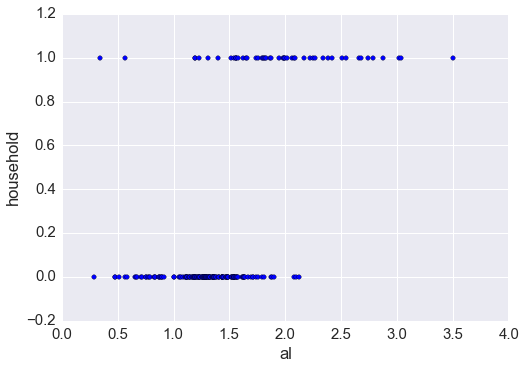Let's draw a regression line, like we did before:

In :
# fit a linear regression model and store the predictions
feature_cols = ['al']
X = glass[feature_cols]
y = glass.household
linreg.fit(X, y)
glass['household_pred'] = linreg.predict(X)

In :
# scatter plot that includes the regression line
plt.scatter(glass.al, glass.household)
plt.plot(glass.al, glass.household_pred, color='red')
plt.xlabel('al')
plt.ylabel('household')

Out:
<matplotlib.text.Text at 0x1a87ddd8>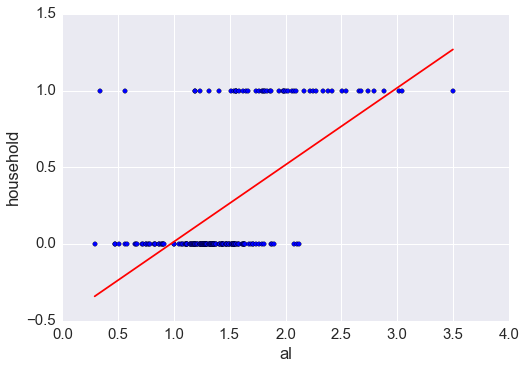If al=3, what class do we predict for household? 1

If al=1.5, what class do we predict for household? 0

We predict the 0 class for lower values of al, and the 1 class for higher values of al. What's our cutoff value? Around al=2, because that's where the linear regression line crosses the midpoint between predicting class 0 and class 1.

Therefore, we'll say that if household_pred >= 0.5, we predict a class of 1, else we predict a class of 0.

In :
# understanding np.where
import numpy as np
nums = np.array([5, 15, 8])

# np.where returns the first value if the condition is True, and the second value if the condition is False
np.where(nums > 10, 'big', 'small')

Out:
array(['small', 'big', 'small'],
dtype='|S5')
In :
# transform household_pred to 1 or 0
glass['household_pred_class'] = np.where(glass.household_pred >= 0.5, 1, 0)
glass.head()

Out:
ri na mg al si k ca ba fe glass_type ri_pred household household_pred household_pred_class
id
22 1.51966 14.77 3.75 0.29 72.02 0.03 9.00 0 0.00 1 1.521227 0 -0.340495 0
185 1.51115 17.38 0.00 0.34 75.41 0.00 6.65 0 0.00 6 1.521103 1 -0.315436 0
40 1.52213 14.21 3.82 0.47 71.77 0.11 9.57 0 0.00 1 1.520781 0 -0.250283 0
39 1.52213 14.21 3.82 0.47 71.77 0.11 9.57 0 0.00 1 1.520781 0 -0.250283 0
51 1.52320 13.72 3.72 0.51 71.75 0.09 10.06 0 0.16 1 1.520682 0 -0.230236 0
In :
# plot the class predictions
plt.scatter(glass.al, glass.household)
plt.plot(glass.al, glass.household_pred_class, color='red')
plt.xlabel('al')
plt.ylabel('household')

Out:
<matplotlib.text.Text at 0x1a8af550>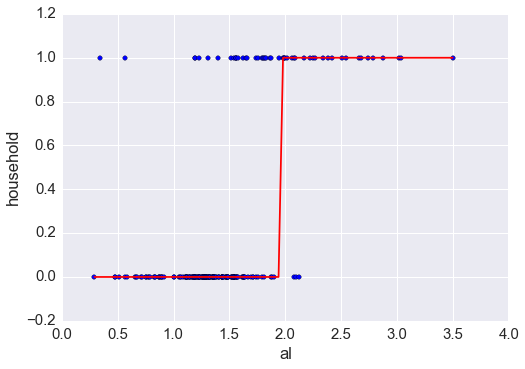## Part 3: Using Logistic Regression Instead¶

Logistic regression can do what we just did:

In :
# fit a logistic regression model and store the class predictions
from sklearn.linear_model import LogisticRegression
logreg = LogisticRegression(C=1e9)
feature_cols = ['al']
X = glass[feature_cols]
y = glass.household
logreg.fit(X, y)
glass['household_pred_class'] = logreg.predict(X)

In :
# plot the class predictions
plt.scatter(glass.al, glass.household)
plt.plot(glass.al, glass.household_pred_class, color='red')
plt.xlabel('al')
plt.ylabel('household')

Out:
<matplotlib.text.Text at 0x1ace2080>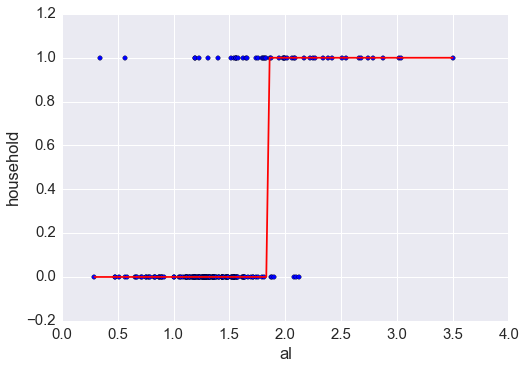What if we wanted the predicted probabilities instead of just the class predictions, to understand how confident we are in a given prediction?

In :
# store the predicted probabilites of class 1
glass['household_pred_prob'] = logreg.predict_proba(X)[:, 1]

In :
# plot the predicted probabilities
plt.scatter(glass.al, glass.household)
plt.plot(glass.al, glass.household_pred_prob, color='red')
plt.xlabel('al')
plt.ylabel('household')

Out:
<matplotlib.text.Text at 0x1accc550>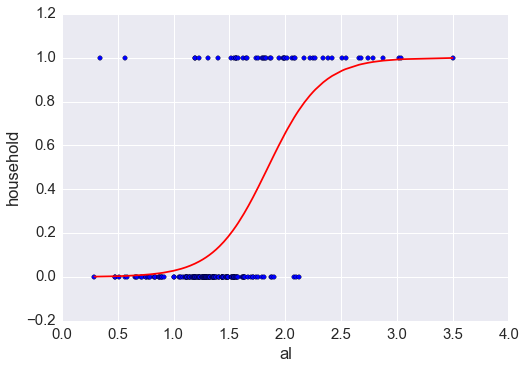In :
# examine some example predictions
print logreg.predict_proba(1)
print logreg.predict_proba(2)
print logreg.predict_proba(3)

[[ 0.97161726  0.02838274]]
[[ 0.34361555  0.65638445]]
[[ 0.00794192  0.99205808]]


The first column indicates the predicted probability of class 0, and the second column indicates the predicted probability of class 1.

## Part 4: Probability, odds, e, log, log-odds¶

$$probability = \frac {one\ outcome} {all\ outcomes}$$$$odds = \frac {one\ outcome} {all\ other\ outcomes}$$

Examples:

• Dice roll of 1: probability = 1/6, odds = 1/5
• Even dice roll: probability = 3/6, odds = 3/3 = 1
• Dice roll less than 5: probability = 4/6, odds = 4/2 = 2
$$odds = \frac {probability} {1 - probability}$$$$probability = \frac {odds} {1 + odds}$$
In :
# create a table of probability versus odds
table = pd.DataFrame({'probability':[0.1, 0.2, 0.25, 0.5, 0.6, 0.8, 0.9]})
table['odds'] = table.probability/(1 - table.probability)
table

Out:
probability odds
0 0.10 0.111111
1 0.20 0.250000
2 0.25 0.333333
3 0.50 1.000000
4 0.60 1.500000
5 0.80 4.000000
6 0.90 9.000000

What is e? It is the base rate of growth shared by all continually growing processes:

In :
# exponential function: e^1
np.exp(1)

Out:
2.7182818284590451

What is a (natural) log? It gives you the time needed to reach a certain level of growth:

In :
# time needed to grow 1 unit to 2.718 units
np.log(2.718)

Out:
0.99989631572895199

It is also the inverse of the exponential function:

In :
np.log(np.exp(5))

Out:
5.0
In :
# add log-odds to the table
table['logodds'] = np.log(table.odds)
table

Out:
probability odds logodds
0 0.10 0.111111 -2.197225
1 0.20 0.250000 -1.386294
2 0.25 0.333333 -1.098612
3 0.50 1.000000 0.000000
4 0.60 1.500000 0.405465
5 0.80 4.000000 1.386294
6 0.90 9.000000 2.197225

## Part 5: What is Logistic Regression?¶

Linear regression: continuous response is modeled as a linear combination of the features:

$$y = \beta_0 + \beta_1x$$

Logistic regression: log-odds of a categorical response being "true" (1) is modeled as a linear combination of the features:

$$\log \left({p\over 1-p}\right) = \beta_0 + \beta_1x$$

This is called the logit function.

Probability is sometimes written as pi:

$$\log \left({\pi\over 1-\pi}\right) = \beta_0 + \beta_1x$$

The equation can be rearranged into the logistic function:

$$\pi = \frac{e^{\beta_0 + \beta_1x}} {1 + e^{\beta_0 + \beta_1x}}$$

In other words:

• Logistic regression outputs the probabilities of a specific class
• Those probabilities can be converted into class predictions

The logistic function has some nice properties:

• Takes on an "s" shape
• Output is bounded by 0 and 1

We have covered how this works for binary classification problems (two response classes). But what about multi-class classification problems (more than two response classes)?

• Most common solution for classification models is "one-vs-all" (also known as "one-vs-rest"): decompose the problem into multiple binary classification problems
• Multinomial logistic regression can solve this as a single problem

## Part 6: Interpreting Logistic Regression Coefficients¶

In :
# plot the predicted probabilities again
plt.scatter(glass.al, glass.household)
plt.plot(glass.al, glass.household_pred_prob, color='red')
plt.xlabel('al')
plt.ylabel('household')

Out:
<matplotlib.text.Text at 0x1b302a58>In :
# compute predicted log-odds for al=2 using the equation
logodds = logreg.intercept_ + logreg.coef_ * 2
logodds

Out:
array([ 0.64722323])
In :
# convert log-odds to odds
odds = np.exp(logodds)
odds

Out:
array([ 1.91022919])
In :
# convert odds to probability
prob = odds/(1 + odds)
prob

Out:
array([ 0.65638445])
In :
# compute predicted probability for al=2 using the predict_proba method
logreg.predict_proba(2)[:, 1]

Out:
array([ 0.65638445])
In :
# examine the coefficient for al
zip(feature_cols, logreg.coef_)

Out:
[('al', 4.1804038614510901)]

Interpretation: A 1 unit increase in 'al' is associated with a 4.18 unit increase in the log-odds of 'household'.

In :
# increasing al by 1 (so that al=3) increases the log-odds by 4.18
logodds = 0.64722323 + 4.1804038614510901
odds = np.exp(logodds)
prob = odds/(1 + odds)
prob

Out:
0.99205808391674566
In :
# compute predicted probability for al=3 using the predict_proba method
logreg.predict_proba(3)[:, 1]

Out:
array([ 0.99205808])

Bottom line: Positive coefficients increase the log-odds of the response (and thus increase the probability), and negative coefficients decrease the log-odds of the response (and thus decrease the probability).

In :
# examine the intercept
logreg.intercept_

Out:
array([-7.71358449])

Interpretation: For an 'al' value of 0, the log-odds of 'household' is -7.71.

In :
# convert log-odds to probability
logodds = logreg.intercept_
odds = np.exp(logodds)
prob = odds/(1 + odds)
prob

Out:
array([ 0.00044652])

That makes sense from the plot above, because the probability of household=1 should be very low for such a low 'al' value.Changing the $\beta_0$ value shifts the curve horizontally, whereas changing the $\beta_1$ value changes the slope of the curve.

## Part 7: Using Logistic Regression with Categorical Features¶

Logistic regression can still be used with categorical features. Let's see what that looks like:

In :
# create a categorical feature
glass['high_ba'] = np.where(glass.ba > 0.5, 1, 0)


Let's use Seaborn to draw the logistic curve:

In :
# original (continuous) feature
sns.lmplot(x='ba', y='household', data=glass, ci=None, logistic=True)

Out:
<seaborn.axisgrid.FacetGrid at 0x1a16bda0>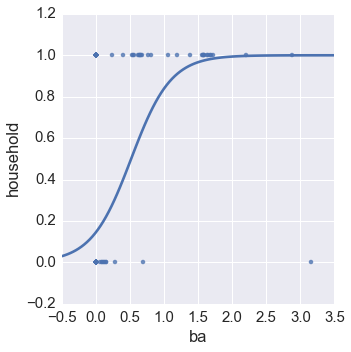In :
# categorical feature
sns.lmplot(x='high_ba', y='household', data=glass, ci=None, logistic=True)

Out:
<seaborn.axisgrid.FacetGrid at 0x1b308e48>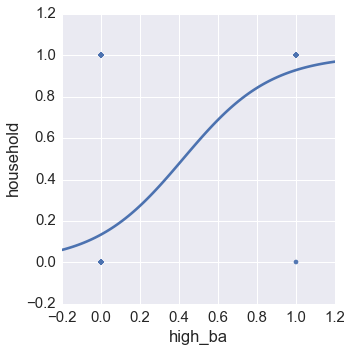In :
# categorical feature, with jitter added
sns.lmplot(x='high_ba', y='household', data=glass, ci=None, logistic=True, x_jitter=0.05, y_jitter=0.05)

Out:
<seaborn.axisgrid.FacetGrid at 0x1bc03710>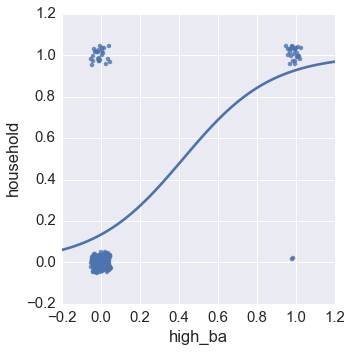In :
# fit a logistic regression model
feature_cols = ['high_ba']
X = glass[feature_cols]
y = glass.household
logreg.fit(X, y)

Out:
LogisticRegression(C=1000000000.0, class_weight=None, dual=False,
fit_intercept=True, intercept_scaling=1, max_iter=100,
multi_class='ovr', penalty='l2', random_state=None,
solver='liblinear', tol=0.0001, verbose=0)
In :
# examine the coefficient for high_ba
zip(feature_cols, logreg.coef_)

Out:
[('high_ba', 4.4273153450187195)]

Interpretation: Having a high 'ba' value is associated with a 4.43 unit increase in the log-odds of 'household' (as compared to a low 'ba' value).

## Part 8: Comparing Logistic Regression with Other Models¶

Advantages of logistic regression:

• Highly interpretable (if you remember how)
• Model training and prediction are fast
• No tuning is required (excluding regularization)
• Features don't need scaling
• Can perform well with a small number of observations
• Outputs well-calibrated predicted probabilities

Disadvantages of logistic regression:

• Presumes a linear relationship between the features and the log-odds of the response
• Performance is (generally) not competitive with the best supervised learning methods
• Can't automatically learn feature interactions